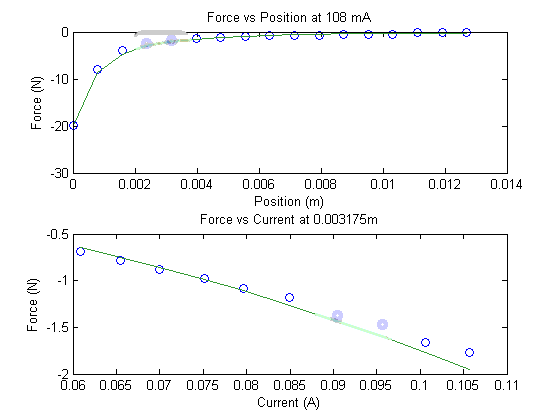# Lab 3a Tips: Electromagnetic Forces

## Pre-Lab

1. Use the values of K, ko, and Ll found during in-lab 2, and not the ones from the pre-lab for the force vs. position and force vs. current plots.
2. You can check your results against the solid lines in the plots below (NOTE: The graph below uses i=108mA).

## In-Lab

1. Measure the applied voltage and current.
2. The hook weighs 50g.
3. Don't let the string rub the table.
4. The electromagnetic force is a function of time. The longer the plunger is in the solenoid while it is on, the stronger the force holding it in. This can make taking measurements of the force difficult ("just a second ago it would hold x grams but now it won't even hold x-20 grams"). The solution is simple. Add weights while the plunger is pulled out. Then briefly move the plunger into contact with the back stop. If it sticks, pull the plunger back out, add more weight, then try again.

## Post-Lab

1. Use the current you measured in lab for the force vs. position prediction plot. (i.e. about 108 mA instead of 200 mA.)